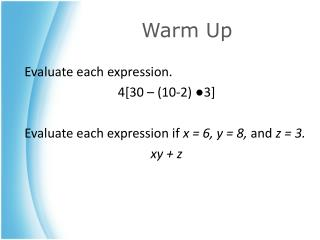Download PresentationWarm Up

# Warm Up

Télécharger la présentation## Warm Up

- - - - - - - - - - - - - - - - - - - - - - - - - - - E N D - - - - - - - - - - - - - - - - - - - - - - - - - - -
##### Presentation Transcript

1. Warm Up Evaluate each expression. 4[30 – (10-2) ●3] Evaluate each expression if x = 6, y = 8, and z = 3. xy + z

2. Open Sentences Prepared by: Ms. Ngo Algebra I Lab

3. Open Sentence • Why learn about open sentence? • Open sentence (mathematical statement) with one or more variables • What meanings do variables and identities carry? (Ex: your name is your identity) • Is an open sentence true or false?

4. Continued… Use a Replacement Set to Solve an Equation Example: Find the solution set for each equation if the replacement set is {4, 5, 6}. Equation Element (member) 6n+ 7 = 37 Open sentence Replacement set

5. Continued… Example: Find the solution set for each equation if the replacement set is {4, 5, 6}. 6n+ 7 = 37 The solution set is {5}.

6. Continued… Find the solution set of an Inequality <, >, ≤, ≥ Example: Find the solution set for 18-y < 10 if the replacement set is {8, 9, 10}.. 18 – y <10 The solution set is {9, 10}.

7. Continued… Find the solution set of an Inequality Example: Find the solution set for 18-y ≤ 10 if the replacement set is {8, 9, 10}.. 18 – y ≤10 The solution set is {8, 9, 10}.

8. Classwork 1) Withyourpartner, pleasework on the followingproblems on « Open Sentences » worksheet. Show all work! #1, 2, 3, 17 2) Exit Paper: Write an example of an equationwith a replacement set and solution set. Don’tforget to label them!